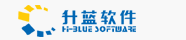Office Automation System

# 把长的数字用逗号隔开显示

March 25,2004
Code Title: Proper Number
Description: If you have a long number to display, then why not make it split the number up correctly with
a comma?

For example, the number 12345678 looks odd, right? Ok, let's apply the Comma() function to that number and
display it like this automatically: 12,345,678 -much cleaner!

By the way, this script supports numbers as long as 15 characters (numbers), so if you have a longer
number than that, we may need to work together to advance this script a bit, but this snippet will work
just fine for your average numbers. Thanx to Dave Cantrell for this advice!
Copy and paste this snippet as-is into your editor:

000000000000000000000000000000000000000000000000000000000000000
<%
Function Comma(str)
If Not(IsNumeric(str)) Or str = 0 Then
Result = 0
ElseIf Len(Fix(str)) < 4 Then
Result = str
Else
Pos = Instr(1,str,".")
If Pos > 0 Then
Dec = Mid(str,Pos)
End if
Res = StrReverse(Fix(str))
LoopCount = 1
While LoopCount <= Len(Res)
TempResult = TempResult + Mid(Res,LoopCount,3)
LoopCount = LoopCount + 3
If LoopCount <= Len(Res) Then
TempResult = TempResult + ","
End If
Wend
Result = StrReverse(TempResult) + Dec
End If
Comma = Result
End Function
%>
<html>
<body>
<%
aLongNumber = "12345678"
%>
An un-formatted number: <%=aLongNumber%><br>
The Comma formatted number: <%=Comma(aLongNumber)%>
</body>
</html>